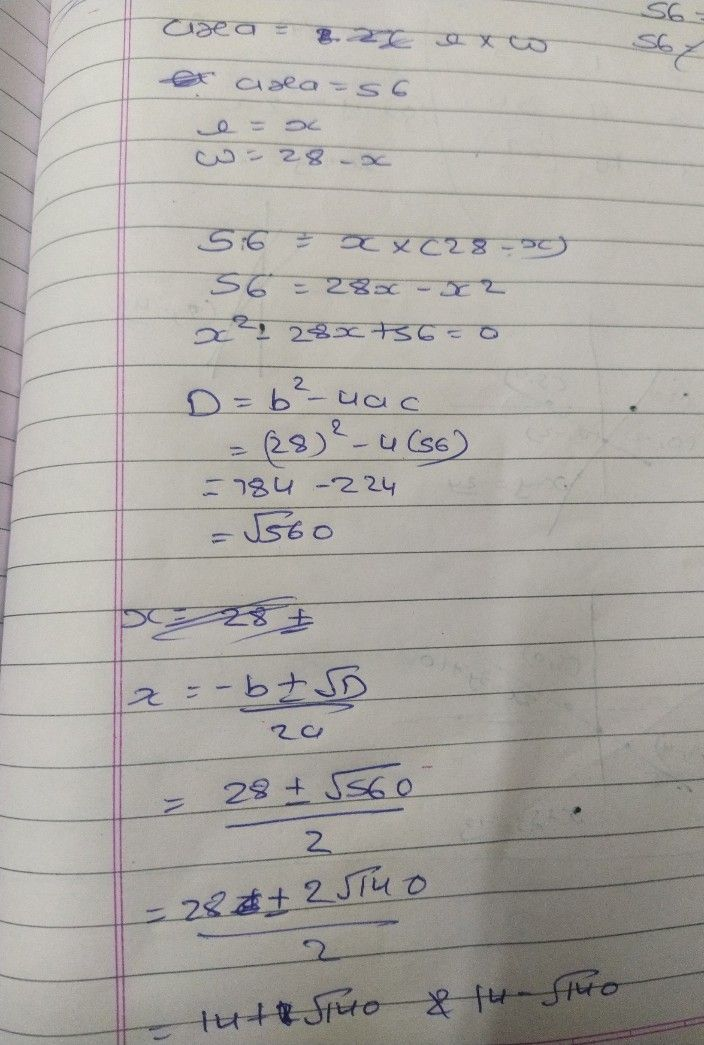Symbol
ProblemLearning Task $3.$ Find the largest area which the farmer can enclose with $56$ m of fencing materials. $28-x$ $x$ $x$ $28-x$